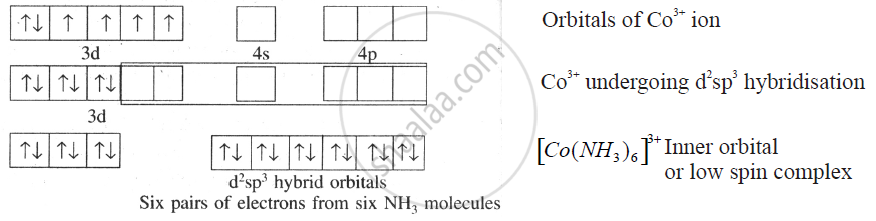HSC Science (Computer Science) 12th Board ExamMaharashtra State Board
Share

# Explain the Geometry of [Co(Nh_3)_6]^(3+) On the Basis of Hybridisation. (Z of Co = 27) - HSC Science (Computer Science) 12th Board Exam - Chemistry

ConceptBonding in Coordination Compounds Valence Bond Theory (VBT)

#### Question

Explain the geometry of [Co(NH_3)_6]^(3+) on the basis of hybridisation. (Z of Co = 27)

#### Solution

Octahedral complex, [Co(NH_3)_6]^(3+). In this complex ion, the oxidation state of cobalt is +3.

It has the electronic configuration as 3d6. This complex involves the d2sp3 hybridisation.The six pairs of electrons, one from each NH3 molecule, occupy the six hybrid orbitals. It proves that complex has octahedral geometry. An absence of unpaired electron makes this complex diamagnetic in nature.

Is there an error in this question or solution?

#### APPEARS IN

2017-2018 (March) (with solutions)
Question 6.2 | 2.00 marks
Solution Explain the Geometry of [Co(Nh_3)_6]^(3+) On the Basis of Hybridisation. (Z of Co = 27) Concept: Bonding in Coordination Compounds - Valence Bond Theory (VBT).
S